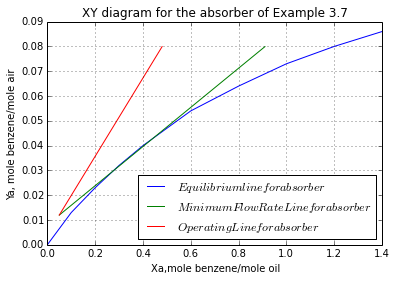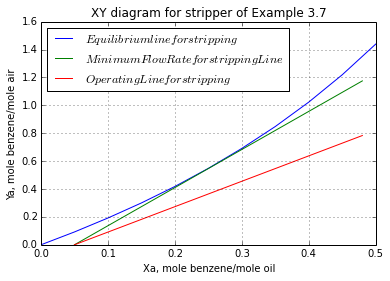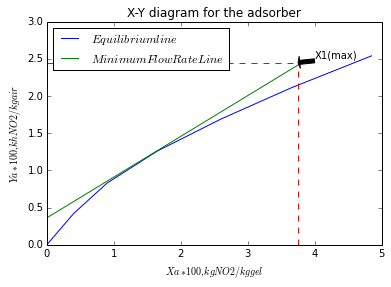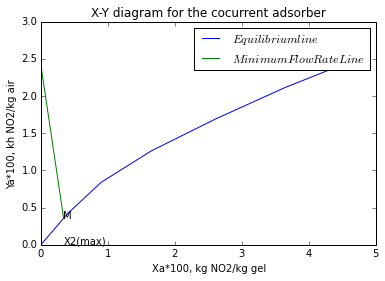# Chapter 3:lnterphase Mass Transfer¶

### Example 3.1,Page number:161¶

In :
#Variable declaration
# a-benzene   b-toluene
T = 300  					# [K]
x_a = 0.4  					# [mole fraction in liquid phase]
# Antoine constants for benzene and toluene are given
# For benzene
A_a = 15.9008
B_a = 2788.51
C_a = -52.36
# For toluene
A_b = 16.0137
B_b = 3096.52
C_b = -53.67

#Calculation

import math

# Using equation 3.5 vapor pressure of component 'a' and 'b'
P_a = math.exp(A_a-(B_a/(T+C_a)))  		# [mm of Hg]
P_b = math.exp(A_b-(B_b/(T+C_b)))  		# [mm of Hg]

P_a = P_a*101.3/760  				# [kPa]
P_b = P_b*101.3/760  				# [kPa]
# Partial pressure of component 'a' and 'b'
p_a = x_a*P_a  					# [kPa]
p_b = (1-x_a)*P_b  				# [kPa]
P_total = p_a+p_b  				# [kPa]

#Result

print"The total equilibrium pressure of the binary system of benzene and toluene is",round(P_total,2)," kPa"

y_a = p_a/P_total  				# [mole fraction in vapor phase]
print"The composition of the vapor in equilibrium is",round(y_a,3)

The total equilibrium pressure of the binary system of benzene and toluene is 8.02  kPa
The composition of the vapor in equilibrium is 0.688


### Example 3.2,Page number:162¶

In :
#Variable declaration
# A-oxygen   B-water
T = 298  					# [K]
H = 4.5*10**4  					# [atm/mole fraction]
P = 1  						# [atm]
row_B = 1000.0 					# [density of water, kg/cubic m]
M_B = 18.0  					# [Molecular mass of water, gram/mole]
M_A = 32.0  					# [,Molecular mass of oxygen, gram/mole]

# Dry air contains 21% oxygen  then p_A = y*P = 0.21 atm
# Therefore using Henry's Law
p_A = 0.21  					# [atm]
x_A = p_A/H  					# [mole fraction in liquid phase]

# Basis: 1L of saturated solution
# For 1 L of very dilute solution of oxygen in water, the total moles of solution, n_t, 	will be approximately equal to the moles of water
n_t = row_B/M_B
# Moles of oxygen in 1L saturated solution is
n_o = n_t*x_A  					# [mole]
# Saturation concentration
c_A = n_o*M_A*1000  				# [mg/L]

#Result

print"The saturation concentration of oxygen in water exposed to dry air at 298 K and 1 atm is",round(c_A,2),"mg/L"

The saturation concentration of oxygen in water exposed to dry air at 298 K and 1 atm is 8.3 mg/L


### Example 3.3,Page number:162¶

In :
#Variable declaration
# a-ammonia   b-air   c-water
T = 300  					# [K]
P = 101.3  					# [kPa]
R = 8.314  					# [cubic m.Pa/mole.K]
V_b = 15.0  					# [cubic m]
m_a = 10.0  					# [kg]
m_c = 45.0  					# [kg]
M_a = 17.0  					# [molecular mass of ammonia, gram/mole
M_c = 18.0  					# [molecular mass of water, gram/mole]

#Calculations

n_b = V_b*P/(R*T)  				# [kmole]
n_a = m_a/M_a  					# [kmole]
n_c = m_c/M_c  					# [kmole]

import math
from scipy.optimize import fsolve

def f12(L_a):
return(((n_a-L_a)/(n_b+n_a-L_a))-(10.51*(0.156+(0.622*(L_a/(n_c+L_a))*(5.765*(L_a/(n_c+L_a))-1)))*(L_a/(n_c+L_a))))
L_a = fsolve(f12,0.3)  # [kmole]

x_a = L_a/(n_c+L_a)
y_a = (n_a-L_a)/(n_b+n_a-L_a)
gammma_a = 0.156+0.622*x_a*(5.765*x_a-1)

#Result

print"At equilibrium the ammonia content of the liquid phase will be",x_a
print"At equilibrium the ammonia content of the gas phase will be",y_a
print"The amount of ammonia absorbed by the water will be",round(L_a,3),"kmole"

At equilibrium the ammonia content of the liquid phase will be 0.144495485596
At equilibrium the ammonia content of the gas phase will be 0.214117393686
The amount of ammonia absorbed by the water will be 0.422 kmole


### Example 3.4,Page number:169¶

In :
#Variable declaration
#  a-ammonia
T  = 300  					# [K]
P = 101.3  					# [kPa]
Kg = 2.75*10**-6  				# [kmole/square m.s.kPa]
m = 1.64
res = 0.85  					# [gas phase resistance]
xa_g = 0.115/100.0  				# [mole fraction of NH3 in liquid phase at a 							point]]

#Calculations

ya_g = 8.0/100.0  					# [mole fraction of NH3 in gas phase at a 							point]
import math
from scipy.optimize import fsolve

Ky = Kg*P  					# [kmole/square m.s]
# Using equation 3.24
ky = Ky/res  					# [kmole/square m.s]
# Using equation 3.21
def f12(kx):
return((m/kx)-(1/Ky)+(1/ky))
kx = fsolve(f12,0.0029)  			# [kmole/square m.s]

# Interfacial concentrations at this particular point in the column, using equation 			(3.15)
ystar_a = m*xa_g
# Using equation 3.12
N_a = Ky*(ya_g-ystar_a)  			# [kmole/square m.s]
# Gas-phase interfacial concentration from equation (3.9)
ya_i = ya_g-(N_a/ky)
# Since the interfacial concentrations lie on the equilibrium line, therefore
xa_i = ya_i/m
# Cross checking the value of N_a
N_a = kx*(xa_i-xa_g)  				# [kmole/square m.s]

#Result

print"The individual liquid film coefficient and gas film coefficient are",round(kx,5),"kmole/square m.s and ",round(ky,6),"kmole/square m.s respectively"

print"The gas phase and liquid phase interfacial concentrations are",round(ya_i,5),"and,",round(xa_i,5),"respectively"

The individual liquid film coefficient and gas film coefficient are 0.00305 kmole/square m.s and  0.000328 kmole/square m.s respectively
The gas phase and liquid phase interfacial concentrations are 0.0136 and, 0.00829 respectively


### Example 3.5,Page number:171¶

In :
#Variable declaration
#  a-ammonia
T = 300  					# [K]
P = 101.3  					# [kPa]
ya_g = 0.6  					# [ammonia concentration in bulk gas]
xa_l = 0.12  					# [ammonia concentration in bulk liquid]
Fl = 3.5*10**-3 	 			# [kmole/square m.s]
Fg = 2*10**-3  					# [kmole/square m.s]

#Calculations

import math
from scipy.optimize import fsolve
# Algebraic solution (a)

def f12(xa_i):
return(1-(1-ya_g)*((1-xa_l)/(1-xa_i))**(Fl/Fg) - 10.51*(0.156+0.622*xa_i*(5.765*xa_i-1))*xa_i)
xa_i = fsolve(f12,0.2)

ya_i = 1-(1-ya_g)*((1-xa_l)/(1-xa_i))**(Fl/Fg)

#Result

print"The local gas and liquid interfacial concentrations are",round(ya_i,3),"and",round(xa_i,3),"respectively"
# Using equation 3.28

N_a = Fg*math.log((1-ya_i)/(1-ya_g))

print"The local ammonia mass-transfer flux is",round(N_a,5),"kmole/square m.s"


The local gas and liquid interfacial concentrations are 0.494 and 0.231 respectively
The local ammonia mass-transfer flux is 0.00047 kmole/square m.s


### Example 3.6,Page number:174¶

In :
#Variable declaration
#  a-methanol   b-water
T = 360.0  # [K]
P = 101.3  # [kPa]
lambda_a = 33.3  # [MJ/kmole]
lambda_b = 41.3  # [MJ/kmole]
Fg = 0.0017  # [kmole/square m.s]
Fl = 0.0149  # [kmole/square m.s]
yag = 0.36  # [bulk gas phase concentration]
xag = 0.20  # [bulk liquid phase concentration]
R = 1.987

#Calculations

import math
from numpy import *
from scipy.optimize import fsolve

sia_ag= 1.0/(1-(lambda_a/lambda_b))
print"sia_g is",sia_ag
#sia_ag =5.155
sia_al = sia_ag
# Therefore equation 3.29 becomes
# yai = 5.155-4.795(4.955/(5.155-xai))**8.765

# Using equation 3.33, 3.34, 3.35
V2 = 18.07  # [cubic cm/mole]
V1 = 40.73  # [cubic cm/mole]
a12 = 107.38  # [cal/mole]
a21 = 469.5  # [cal/mole]

# Solution of simultaneous equation
def F(e):
x1,x2,y1,y2,e4=e

#####SIDE Quantities#####
a=(V1/V2)*math.exp(-a21/(R*e4))           #d2lT
a1=(V2/V1)*math.exp(-a12/(R*e4))             #dl2(T)
b=math.exp(16.2620-3800.0/(e4-47))           #P2(T)
b1=math.exp(16.5938-3644.3/(e4-33))           #P1(T)
c=math.exp(-log(x2+x1*a)-x1*(a1/(x1+x2*a1)-a/(x2+x1*a)))     #gamma_2
d=math.exp(-log(x1+x2*a1)-x1*(a1/(x1+x2*a1)-a/(x2+x1*a)))    #gamma_1
#########################
x1=1-x2
y2=1-y1
y1=5.155-4.795*(4.955/(5.155-x2))**(Fl/Fg)
y1=x1*d*b1/P
y2=x2*c*b/P
return(x1,x2,y1,y2,e4)
# Initial guess
y = fsolve(F,(0.1,0.9,0.2,0.8,300))
xai = y
xbi = y
yai = y
ybi = y
T = y  # [K]

print"yai is",yai
print"ybi is",ybi
print"xai is",xai
print"xbi is",xbi
print"Temperature is",T,"K"
# Local Methanol flux, using equation 3.28
Na = sia_ag*Fg*math.log((sia_ag-yai)/(sia_ag-yag))  # [kmole/square m.s]
# From energy balance
Nb = -(lambda_a/lambda_b)*Na
#Result

print"Local Methanol flux is",round(Na,4)," (APPROX) kmole/square m.s"

sia_g is 5.1625
yai is 0.2
ybi is 0.8
xai is 0.1
xbi is 0.9
Temperature is 300.0 K
Local Methanol flux is 0.0003  (APPROX) kmole/square m.s


### Example 3.7,Page number:183¶

In :
#Variable declaration
# 1-benzene a-absorber s-steams
T = 300  						# [K]
P = 101.3  						# [kPa]
R = 8.314  						# [gas constant]
v = 1  							# [cubic m/s]
# Gas in
y1a = 0.074
# Liquid in
x2a = 0.0476
# Recovery is 85 %
# Calculations for absorber section

#Calculations
import matplotlib
%matplotlib inline
import math
from pylab import *
from numpy import *

V1a = P*v/(R*T)  					# [kmole/s]
# Inert gas molar velocity
Vsa = V1a*(1-y1a)  					# [kmole/s]
Y1a = y1a/(1-y1a)  					# [kmole of benzene/kmole of dry gas]

X2a = x2a/(1-x2a)  					# [kmole of benzene/kmole of oil]
# Since the absorber will recover 85% of the benzene in the entering gas, the 		concentration of the gas leaving it will be
r = 0.85
Y2a = (1-r)*Y1a  					# [kmole of benzene/kmole of dry gas]
# The benzene-wash oil solutions are ideal, and the pressure is low  therefore, Raoult’s 		law applies. From equations 3.1, 3.44, and 3.45
#      yia = 0.136*xia
# or   Yia/(1+Yia) = 0.136*Xia/(1+Xia)

# Data_eqm = [Xia,Yia]
Data_eqm =matrix([[0,0],[0.1,0.013],[0.2,0.023],[0.3,0.032],[0.4,0.04],[0.6,0.054],[0.8,0.064],[1,0.073],[1.2,0.080],[1.4,0.086]])

# Here because of the shape of equilibrium curve, the operating line for minimum oil rate 	must be tangent to curve
# Therefore
# From the curve X1a_max = 0.91
X1a_max = 0.91  					# [kmol benzene/kmol oil]

# For minimum operating line slope is
S = (Y1a-Y2a)/(X1a_max-X2a)  				# [kmol oil/kmol air]
# Therfore
Lsa_min = S*Vsa  					# [kmole oil/s]
Data_minSlope1 = matrix([[X2a,Y2a],[X1a_max,Y1a]])

# For Actual operating line, oil flow rate is twice the minimum
Lsa = 2*Lsa_min  					# [kmole oil/s]
M_oil = 198  						# [molecular weight of oil, gram/mole]

Wsa = Lsa*M_oil  					# [mass flow rate of oil, kg/s]
# Using equation 3.47 to calculate the actual concentration of the liquid phase leaving 	the absorber
X1a = X2a + Vsa*(Y1a-Y2a)/Lsa  				# [kmol benzene/kmol oil]
Data_opline1 = matrix([[X2a,Y2a],[X1a,Y1a]])
figure(1)
a1=plot(Data_eqm[:,0],Data_eqm[:,1],label='$Equilibrium line for absorber$')
a2=plot(Data_minSlope1[:,0],Data_minSlope1[:,1],label='$Minimum Flow Rate Line for absorber$')
a3=plot(Data_opline1[:,0],Data_opline1[:,1],label='$Operating Line for absorber$')
legend(loc='lower right')
plt.grid()
xlabel("Xa,mole benzene/mole oil")
ylabel("Ya, mole benzene/mole air")
title("XY diagram for the absorber of Example 3.7")
show(a1)
show(a2)
show(a3)
# Calculations for stripping section
Lss = Lsa
X2s = X1a
X1s = X2a
Y1s = 0
T = 373  # [K]
# Applying Raoult’s law at this temperature gives us
# yis = 1.77*xis
# Yis/(1+Yis) = 1.77*Xis/(1+Xis)

# Equilibrium data
# Data_equm = [Xis,Yis]
Data_equm = matrix([[0,0],[0.05,0.092],[0.1,0.192],[0.15,0.3],[0.2,0.418],[0.25,0.548],[0.3,0.691],[0.35,0.848],[0.4,1.023],[0.45,1.219],[0.5,1.439]])

# Similar procedure as above is followed
# The operating line for minimum oil rate must be tangent to curve
# Therefore from the curve
Y2s_max = 1.175  					# [kmol benzene/kmol steam]
S = (Y2s_max-Y1s)/(X2s-X1s)  				# [kmole oil/kmole steam]
Vss_min = Lss/S  					# [kmole/s]
Vss = 1.5*Vss_min  					# [kmole/s]
Mss = 18 						# [molecular weight of steam, gram/mole]
Wss = Vss*Mss  						# [kg steam/s]

Data_minSlope2 = matrix([[X1s,Y1s],[X2s,Y2s_max]])

Y2s_act = Y1s + Lss*(X2s-X1s)/Vss  			# [kmol benzene/kmol steam]

Data_opline2 = matrix([[X1s,Y1s],[X2s,Y2s_act]])

figure(2)
a1=plot(Data_equm[:,0],Data_equm[:,1],label='$Equilibrium line for stripping$')
a2=plot(Data_minSlope2[:,0],Data_minSlope2[:,1],label='$Minimum Flow Rate for stripping Line$')
a3=plot(Data_opline2[:,0],Data_opline2[:,1],label='$Operating Line for stripping$')
#legend("Equilibrium line for stripping","Minimum Flow Rate for stripping Line","Operating Line #for stripping")
legend(loc='upper left')
xlabel("Xa, mole benzene/mole oil")
ylabel("Ya, mole benzene/mole air")
title("XY diagram for stripper of Example 3.7")
plt.grid()
show(a1)
show(a2)
show(a3)
#Result

print"The oil circulation rate and steam rate required for the operation is",round(Wsa,2),"kg/s",round(Wss,3)," kg steam/s respectively"The oil circulation rate and steam rate required for the operation is 1.18 kg/s 0.059  kg steam/s respectively


### Example 3.8,Page number:190¶

In :
import matplotlib
%matplotlib inline
from numpy import *
from pylab import *
#Variable declaration
# 1-Nitrogen dioxide  2-air
T = 298  					# [K]
P = 101.3  					# [kPa]
y1 = 0.015
V1 = 0.5  					# [mass flow rate of the gas entering the 						adsorber, kg/s]
M1 = 46  					# [gram/mole]
M2 = 29  					# [gram/mole]

#Calculations

# Data_eqm1 = [P1 m] (where 'P1' is Partial pressure of NO2 in mm of Hg, 'm' is solid 	concentration in kg NO2/kg gel)
Data_eqm1 = matrix([[0,0],[2,0.4],[4,0.9],[6,1.65],[8,2.60],[10,3.65],[12,4.85]])

Y1 = y1*M1/((1-y1)*M2)  			# [kg NO2/kg air]
# For 85% removal of the NO2,
Y2 = 0.15*Y1  					# [kg NO2/kg air]
# Since the entering gel is free of NO2,
X2 = 0

Data_eqm =matrix([[0,0],[0.4,0.42],[0.9,0.83],[1.65,1.26],[2.6,1.69],[3.65,2.11],[4.85,2.54]])

# The operating line for minimum slope is tangent to curve, from which we get
X1_max = 0.0375  				# [kg NO2/kg gel]

wb1 = 1/(1+Y1)
Vs = V1*wb1  					# [mass velocity of the air, kg/s]
Ls_min = Vs*(Y1-Y2)/(X1_max-X2)  		# [kg gel/s]
Data_minSlope = matrix([[X2,Y2],[X1_max,Y1]])*100
# Operating line
Ls = 2*Ls_min  					# [kg gel/s]

X1 = X2 + Vs*(Y1-Y2)/Ls  			# [kg NO2/kg gel]

#Result

a1=plot(Data_eqm[:,0],Data_eqm[:,1],label='$Equilibrium line$')
a2=plot(Data_minSlope[:,0],Data_minSlope[:,1],label='$Minimum Flow Rate Line$')
a3=plot((3.75,3.75),(0,2.45),'--')
a4=plot((0,3.75),(2.45,2.45),'r--')
legend(loc='upper left')
xlabel("$Xa*100, kg NO2/kg gel$")
ylabel("$Ya*100, kh NO2/kg air$")
annotate("X1(max)", xy=(3.75, 2.45), xytext=(4,2.5),
arrowprops=dict(facecolor='black', shrink=0.01),
)
show(a1)
show(a2)
show(a3)
show(a4)
print"Mass flow rate of the and the composition of the gel leaving the absorber are",round(Ls,3),"kg gel/s and",round(X1,4),"kg No2/kg gel"Mass flow rate of the and the composition of the gel leaving the absorber are 0.535 kg gel/s and 0.0187 kg No2/kg gel


### Example 3.9,Page number:194¶

In :
#Variable declaration
# 1-Nitrogen dioxide  2-air
# From Example 3.8
Y1 = 0.0242  					# [kg NO2/kg air]
Y2 = 0.0036  					# [kg NO2/kg air]
Vs = 0.488  					# [kg air/s]
M1 = 46  					# [gram/mole]
M2 = 29  					# [gram/mole]
# However here
import matplotlib
%matplotlib inline
import math
from pylab import *
from numpy import *
X1 = 0

#Calculations

# Data_eqm1 = [P1 m] (where 'P1' is Partial pressure of NO2 in mm of Hg, 'm' is solid 	concentration in kg NO2/kg gel)
Data_eqm1 =matrix([[0,0],[2,0.4],[4,0.9],[6,1.65],[8,2.60],[10,3.65],[12,4.85]])

# The equilibrium data are converted to mass ratios as follows:
# Yi = P1/(760-P1)*46/29 (kg NO2/kg air)  Xi = m/100 (kg NO2/kg gel)
# Equilibrium data
# Data_eqm = [Xi*100 Yi*100]

Data_eqm=matrix([[0,0],[0.4,0.4185242],[0.9,0.8392629],[1.65,1.2622336],[2.6,1.6874541],[3.65,2.1149425],[4.85,2.5447169]])
# From the intersection of the minimum operating line and equilibrium curve
X2_max = 0.0034  				# [kg NO2/kg gel]
S = (Y1-Y2)/(X1-X2_max)  			# [kg gel/kg air]
Ls_min = -S*Vs  				# [kg/s]

Ls = 2*Ls_min  					# [kg/s]
Data_minSlope =matrix([[X1,Y1],[X2_max,Y2]])*100

#Result

a1=plot(Data_eqm[:,0],Data_eqm[:,1],label='$Equilibrium line$')
a2=plot(Data_minSlope[:,0],Data_minSlope[:,1],label='$Minimum Flow Rate Line$')
legend(loc='upper right')
xlabel("Xa*100, kg NO2/kg gel")
ylabel("Ya*100, kh NO2/kg air")
title('X-Y diagram for the cocurrent adsorber')
annotate("M", xy=(0.34,0.36), xytext=(0.34,0.36)
)
annotate("X2(max)", xy=(0.34,0.0), xytext=(0.34,0.01)
)
show(a1)
show(a2)
print"The mass velocity of the silica gel required for cocurrent operation is",round(Ls,2),"kg/s which is 11 times that required for countercurrent operation"The mass velocity of the silica gel required for cocurrent operation is 5.91 kg/s which is 11 times that required for countercurrent operation


### Example 3.10,Page number:199¶

In :
#Variable declaration
# From Example 3.7
X2a = 0.05
X0 = X2a  				# [kmole benzene/kmole oil]
Y2a = 0.012
Y1 = Y2a  				# [kmole benzene/kmole dry gas]
X1a = 0.480
Xn = X1a  				# [kmole benzene/kmole oil]
Y1a = 0.080
Yn1 = Y1a  				# [kmole benzene/kmole dry gas]
# Ideal stages for absorber section

m = 0.097  				# [mole of oil/mole of dry gas]
Lsa = 0.006  				# [kmole/s]
Vsa = 0.038  				# [kmole/s]

#Calculations

A = Lsa/(m*Vsa)  			# [Absorption factor]
import math
# From equation 3.54 by Kremser equation
Nk = math.log((((Yn1-m*X0)*(1-1/A))/(Y1-m*X0))+1/A)/(math.log(A))

# Ideal stages from graph
# Stair case construction is being made between equilibrium curve and operating line from 	piont X2a,Y2a to X1a,Y1a
# A more precise estimate of stages
# From figure 3.25 or from graph made for absorber in Example 3.7
Xa = 0.283
Xb = 0.480
Xc = 0.530
Na = 3+(Xb-Xa)/(Xc-Xa)
print"ABSORBER"
print"The number of ideal stages from graph in the absorber is",round(Na,1)
print"Number of ideal stages from Kremser equation in the absorber is",round(Nk,2)
# Ideal satges for stripping section
X2s = 0.480
X0 = X2s  				# [kmol benzene/kmol oil]
Y2s = 0.784
Y1 = Y2s  				# [kmol benzene/kmol steam]
X1s = 0.05
Xn = X1s  				# [kmol benzene/kmol oil]
Y1s = 0
Yn1 = Y1s  				# [kmol benzene/kmol steam]

# Similarly here also stair case construction is being made between equilibrium curve and 	operating line from piont X0,Y1 to Xn,Yn1
# A more precise estimate of stages
# From figure 3.26 or from graph made for stripping section in Example 3.7
Ns = 5+(0.070-0.050)/(0.070-0.028)

#Result
print"STRIPPER"
print"The number of ideal stages from graph in the stripping section is",round(Ns,1)

ABSORBER
The number of ideal stages from graph in the absorber is 3.8
Number of ideal stages from Kremser equation in the absorber is 3.16
STRIPPER
The number of ideal stages from graph in the stripping section is 5.5